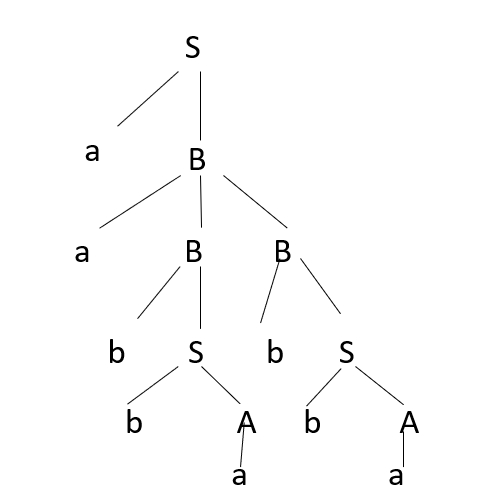# Construct the derivation tree for the string aabbabba.

## Problem

Derive a derivation tree for the string aabbabba for the given context free grammar (CFG) −

S->aB|bA
A->a|aS|bAA
B->b|bS|aBB

## Solution

Derivation is a sequence of production rules, which is used to get input strings.

### Derivation tree

It is a graphical representation for derivation of given production rules for a given CFG. It is also called as a parse tree.

### Properties

The derivation tree contains some properties, which are as follows −

• The root node is always a node indicating the start symbol.
• The derivation is read from left to right.
• The leaf node is always terminal nodes.
• The interior nodes are always the non-terminal nodes.

The given CFG is as follows −

S->aB|bA
A->a|aS|bAA
B->b|bS|aBB

To draw a parse tree first try to obtain derivation for the string aabbabba

Let’s take leftmost derivation as shown below −

S-> aB
->aaBB
->aabSB
->aabbAB
->aabbaB
->aabbabS
->aabbabbA
->aabbabba which is our final string

Now, construct the derivation tree for the given grammar.

If the grammar is generating more than one type of tree, then we call the given grammar ambiguous grammar.